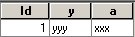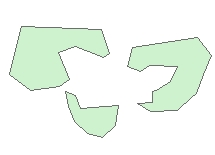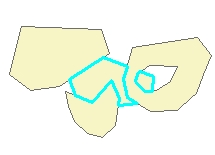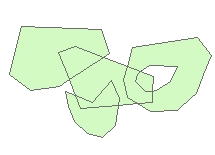Go to ET GeoWizards 12.x User Guide

Go to ToolBox Implementation

Merges two polygon data sets. The result does not contain overlaps

Inputs:

• Base layer - a polygon layer that will keep all attribute fields
• Merge layer - a polygon layer that will be merged to the Base layer.
• Priority of the Merge layer as described below
• Priority - "Erase" - the polygons from the Base layer are erased with the polygons of the Merge layer
• Priority - "Low" -  only the polygons (or portions of them) from the Merge layer that do not overlap with these of the Base layer are added to the new layer
• Priority - "Standard" - Creates intersections where the polygons from the Merge layer  intersect these from the base layer. The intersection polygons carry the attributes of the corresponding polygons from both layers
• Priority - "High"  - The polygons from the Merge layer are entirely preserved. Only these polygons (or portions of them) from the base layer that do not overlap with the polygons from the Merge layer are added to the output.

Outputs:

• New  Polygon feature class
• No overlaps present. The polygons from the Base and the Merge layers are overlaid depending the priority of the Merge layer
• All the attributes of the Base layer are preserved
• Only the attributes in fields with the same name and type as these from the Base layer are preserved for the features from the Merge layer. Exception makes priority "Standard" which copies the attributes from the merge layer as well.
• Base layer always have a Priority "Standard"

Examples:

 Input LayersBase layer tableMerge layer tableResult: Priority of "-1" (Erase)Result tableResult: Priority of "0" (Low)Result tableResult: Priority of "1" (Standard)Result tableResult: Priority of "2" (High)Result tableCommand line syntax

ET_GPAdvancedMerge <input_dataset> <merge_dataset> <out_feature class> <merge_priority>  {fuzzy_tolerance}

Parameters

Expression Explanation
<input_dataset> A Polygon feature class or feature layer
<merge_dataset> A Polygon feature class or feature class.
NOTE: The spatial references of <merge_dataset> and the <input_dataset> must have the same Geographic Coordinate System
<out_feature_class> A String - the full name of the output feature class (A feature class with the same full name should not exist)
<merge_priority> Required. A string representing the priority of the polygons to be merged
• Priority of Erase - the polygons from the Base dataset are erased with the polygons of the Merge dataset
• Priority of Low -  only the polygons (or portions of them) from the Merge dataset that do not overlap with these of the Base layer are added to the new dataset
• Priority of Standard - Creates intersections where the polygons from the Merge dataset intersect these from the base layer. The intersection polygons carry the attributes of the corresponding polygons from both datasets
• Priority of High - The polygons from the Merge dataset are entirely preserved. Only these polygons (or portions of them) from the base dataset that do not overlap with the polygons from the Merge dataset are added to the output.
{fuzzy_tolerance} A Double representing  the Fuzzy tolerance (in the units of the input dataset) to be used

Scripting syntax

ET_GPAdvancedMerge (input_dataset, merge_dataset, out_feature class, merge_priority, fuzzy_tolerance)

Example Python script:

 import arcpyarcpy.ImportToolbox("C:/Program Files/ET SpatialTechniques/ET GeoWizards 11.0 for ArcGIS 10.1/ET GeoWizards.tbx")input_dataset = "C:\\data\\pg1.shp"merge_dataset = "C:\\data\\fgdb_test.gdb\\pg2"result = "C:\\data\\fgdb_test.gdb\\erased"arcpy.gp.toolbox = "C:/Program Files/ET SpatialTechniques/ET GeoWizards 11.0 for ArcGIS 10.1/ET GeoWizards.tbx");arcpy.ET_GPAdvancedMerge(input_dataset, merge_datase, result, "Erase", "0.003426878")